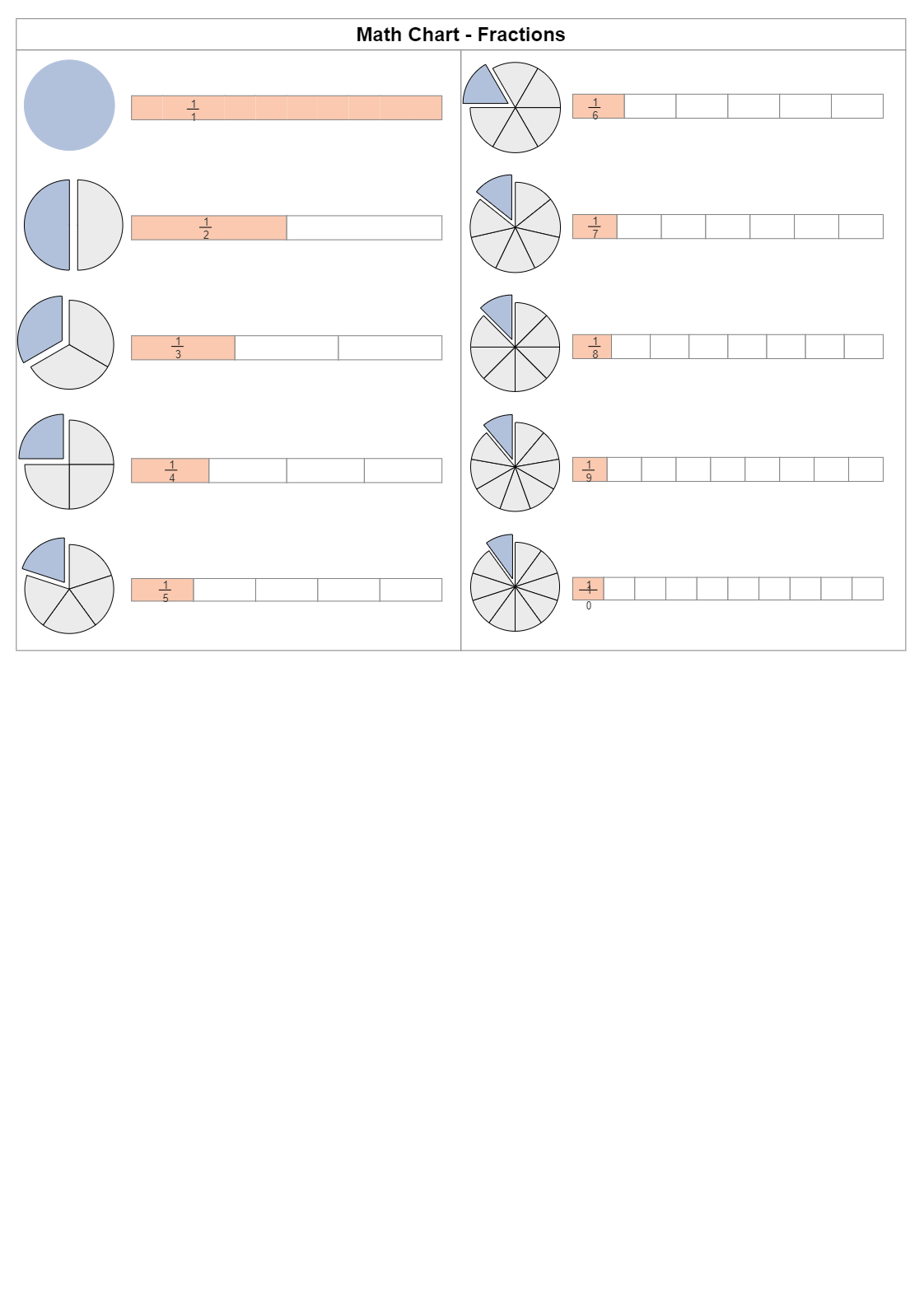# Fraction Chart

Use Template9
0
Report
Publish time：06-11-2021
Tag:
science diagram
Mathematics Diagram
In mathematics, a fraction chart represents equal parts of a whole or a collection. Fraction of a whole: when we divide a total into equal parts, each part is a fraction of the whole. As depicted in the following chart, a fraction tells the mathematics student how many parts of a whole we have. Students can recognize a fraction by the slash that is written between the two numbers. The size of fractions can also be compared to find the smallest or the largest fraction. Teachers can use EdrawMax to create a fraction chart for the tests and distribute it among the peers. The following fraction chart diagram shows fraction as a whole; the fraction has half, fraction as a third, fraction as a fourth, and so on. This lets the students learn more about fractions.
CreatorKiraaaaPost
Recommended Templates